## Summary and Analysis of Extension Program Evaluation in R

Salvatore S. Mangiafico

# Two-way Repeated Ordinal Regression with CLMM

A two-way repeated ordinal analysis of variance can address an experimental design with two independent variables, each of which is a factor variable, plus a blocking variable.  The main effect of each independent variable can be tested, as well as the effect of the interaction of the two factors.

The example here looks at students’ knowledge scores for three speakers across two different times.  Because we know which student has each score at each time, we will use the Student as a blocking variable, with the suspicion that one student might have consistently lower knowledge than another student.  If we hadn’t recorded this information about students, we could use a two-way ordinal regression.

As a matter of practical interpretation, we may not care about the absolute scores for each of the three speakers, only if the knowledge scores for students increased from Time 1 to Time 2.  At Time 1, students’ knowledge scores are lower for Piglet that for the other two speakers.  This isn’t the fault of Piglet; he is just speaking about a topic that the students have little knowledge of.

The clmm function can specify more complex models with multiple independent variables of different types, but this book will not explore more complex examples.

The p-values for the main and interaction effects can be determined with the Anova function from RVAideMemoire, which produces an analysis of deviance table for these effects.  In addition, a p-value for the model as a whole will be determined, along with a pseudo R-squared for the model as a whole.

Post-hoc analysis to determine which groups are different can be conducted on each significant main effect and on the interaction effect if it is significant.

### Packages used in this chapter

The packages used in this chapter include:

•  psych

•  FSA

•  lattice

•  ggplot2

•  ordinal

•  car

•  RVAideMemoire

•  lsmeans

•  multcompView

•  plyr

•  boot

•  rcompanion

The following commands will install these packages if they are not already installed:

if(!require(psych)){install.packages("psych")}
if(!require(FSA)){install.packages("FSA")}
if(!require(lattice)){install.packages("lattice")}
if(!require(ggplot2)){install.packages("ggplot2")}
if(!require(ordinal)){install.packages("ordinal")}
if(!require(car)){install.packages("car")}
if(!require(RVAideMemoire)){install.packages("RVAideMemoire")}
if(!require(lsmeans)){install.packages("lsmeans")}
if(!require(multcompView)){install.packages("multcompView")}
if(!require(plyr)){install.packages("plyr")}
if(!require(boot)){install.packages("boot")}
if(!require(rcompanion)){install.packages("rcompanion")}

### Two-way repeated ordinal regression example

Input =("
Speaker  Student  Time  Likert
Pooh        a       1       3
Pooh        b       1       5
Pooh        c       1       4
Pooh        d       1       4
Pooh        e       1       4
Pooh        f       1       3
Pooh        g       1       4
Pooh        h       1       4

Pooh        a       2       4
Pooh        b       2       5
Pooh        c       2       5
Pooh        d       2       5
Pooh        e       2       5
Pooh        f       2       4
Pooh        g       2       5
Pooh        h       2       5

Piglet      i       1       1
Piglet      j       1       2
Piglet      k       1       1
Piglet      l       1       1
Piglet      m       1       1
Piglet      n       1       2
Piglet      o       1       3
Piglet      p       1       1

Piglet      i       2       2
Piglet      j       2       4
Piglet      k       2       2
Piglet      l       2       1
Piglet      m       2       2
Piglet      n       2       2
Piglet      o       2       4
Piglet      p       2       2

Eeyore      q       1       4
Eeyore      r       1       5
Eeyore      s       1       4
Eeyore      t       1       4
Eeyore      u       1       4
Eeyore      v       1       4
Eeyore      w       1       4
Eeyore      x       1       4

Eeyore      q       2       5
Eeyore      r       2       4
Eeyore      s       2       4
Eeyore      t       2       4
Eeyore      u       2       3
Eeyore      v       2       4
Eeyore      w       2       4
Eeyore      x       2       4
")

### Change Time into a factor variable

Data\$Time = factor(Data\$Time)

### Order levels of the factor; otherwise R will alphabetize them

Data\$Speaker = factor(Data\$Speaker,
levels=unique(Data\$Speaker))

### Create a new variable which is the likert scores as an ordered factor

Data\$Likert.f = factor(Data\$Likert,
ordered = TRUE)

###  Check the data frame

library(psych)

str(Data)

summary(Data)

### Remove unnecessary objects

rm(Input)

#### Summarize data treating Likert scores as factors

xtabs( ~ Time + Likert.f + Speaker,
data = Data)

Likert.f
Time 1 2 3 4 5
1 0 0 2 5 1
2 0 0 0 2 6

, , Speaker = Piglet

Likert.f
Time 1 2 3 4 5
1 5 2 1 0 0
2 1 5 0 2 0

, , Speaker = Eeyore

Likert.f
Time 1 2 3 4 5
1 0 0 0 7 1
2 0 0 1 6 1

#### Bar plots by group

library(lattice)

histogram(~ Likert.f | Speaker,
data=Data,
layout=c(1,3)      #  columns and rows of individual plots
)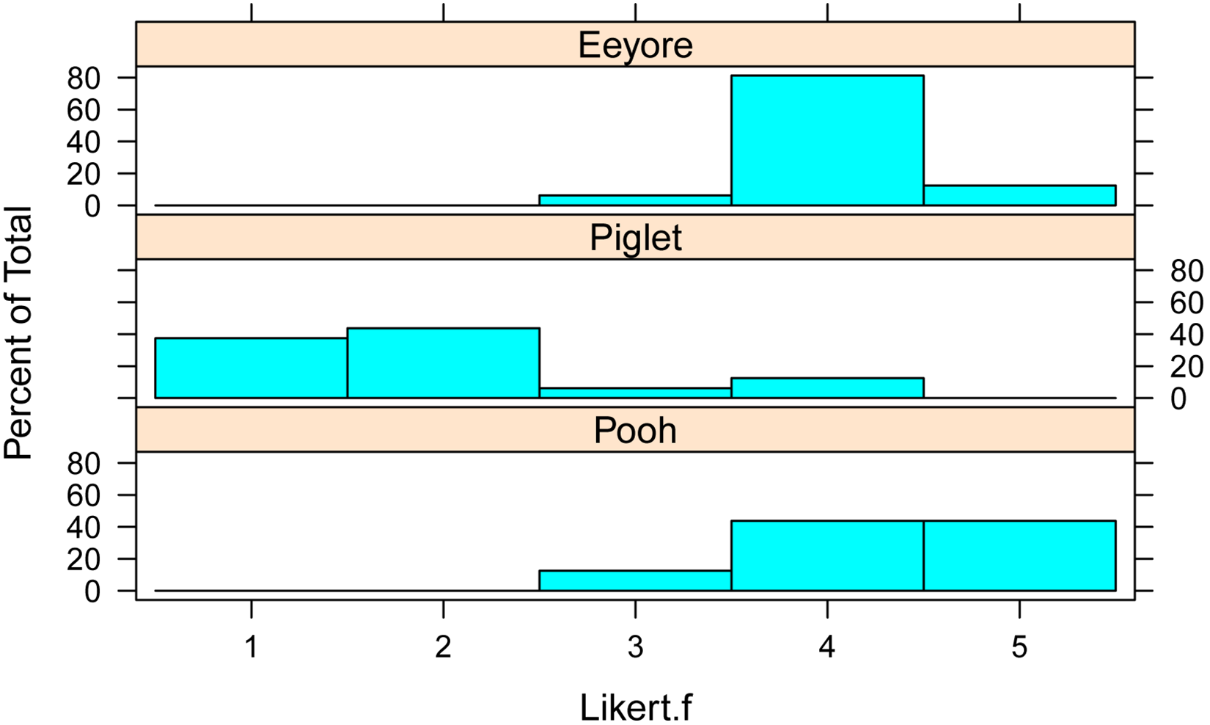library(lattice)

histogram(~ Likert.f | Time,
data=Data,
layout=c(1,2)      #  columns and rows of individual plots
)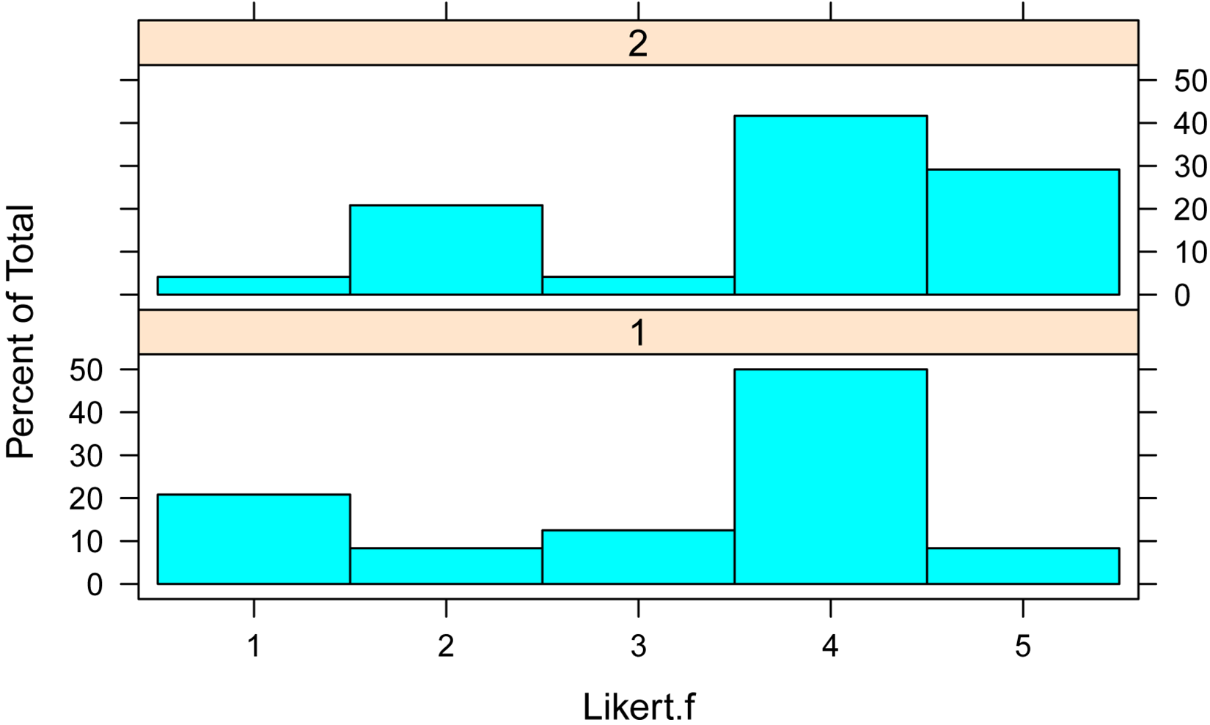library(lattice)

histogram(~ Likert.f | Time + Speaker,
data=Data,
layout=c(2,3)      #  columns and rows of individual plots
)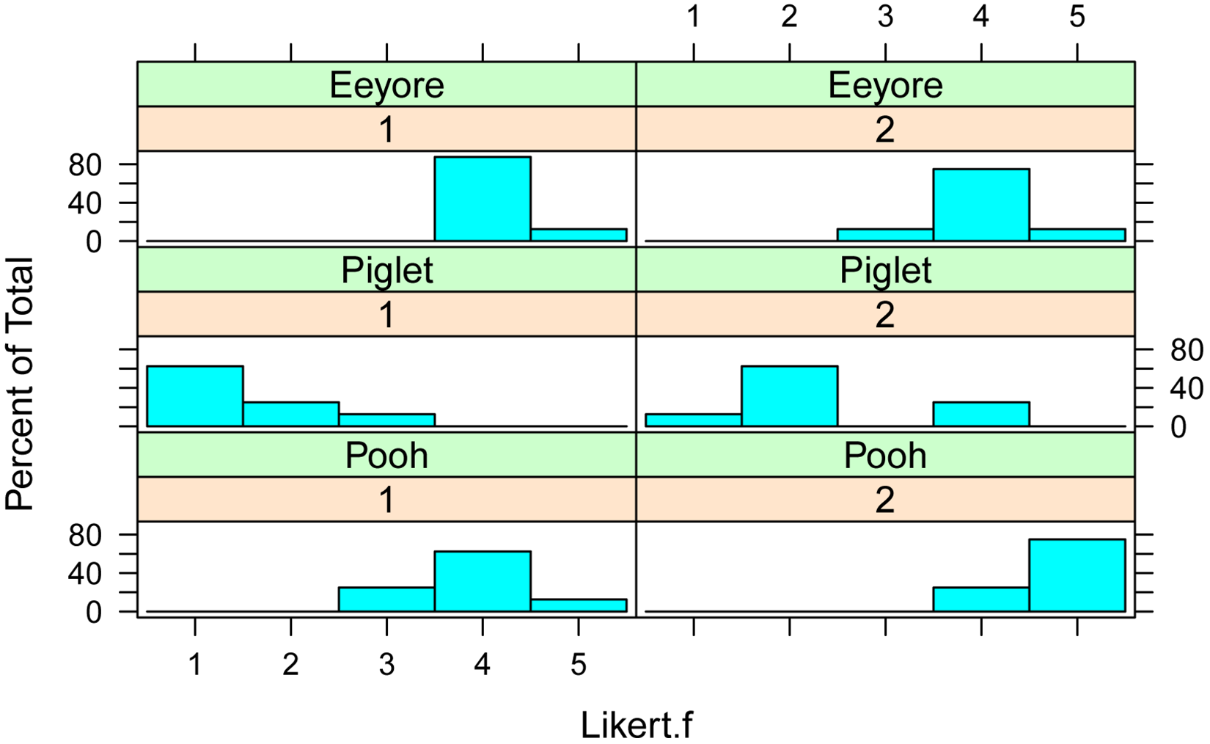#### Summarize data treating Likert scores as numeric

library(FSA)

Summarize(Likert ~ Speaker + Time,
data=Data,
digits=3)

Speaker Time n nvalid  mean    sd min   Q1 median  Q3 max percZero
1    Pooh    1 8      8 3.875 0.641   3 3.75      4 4.0   5        0
2  Piglet    1 8      8 1.500 0.756   1 1.00      1 2.0   3        0
3  Eeyore    1 8      8 4.125 0.354   4 4.00      4 4.0   5        0
4    Pooh    2 8      8 4.750 0.463   4 4.75      5 5.0   5        0
5  Piglet    2 8      8 2.375 1.061   1 2.00      2 2.5   4        0
6  Eeyore    2 8      8 4.000 0.535   3 4.00      4 4.0   5        0

#### Plot Likert scores before and after

Since we are most interested in pairing scores from Time 1 to Time 2, we can plot the data in a way that pairs points.

Points above and to left of the blue line indicate cases in which the score for Time 2 is greater than that for Time 1.

Note that the data must be ordered by Student and Speaker for this code to plot the data correctly.  That is, the first observation for Time==1 must be the same student and speaker as the first observation for Time==2

Also note that the points on the plot are jittered so that multiple points with the same values will be visible.

Time.1  = Data\$Likert[Data\$Time==1]
Time.2  = Data\$Likert[Data\$Time==2]
Speaker = Data\$Speaker[Data\$Time==2]

plot(Time.1, jitter(Time.2),
pch = 16,
cex = 1,
col = Speaker,
xlab="Time 1",
ylab="Time 2")

abline(0,1, col="blue", lwd=2)

legend('bottomright',
legend = unique(Speaker),
col = 1:3,
cex = 1,
pch = 16)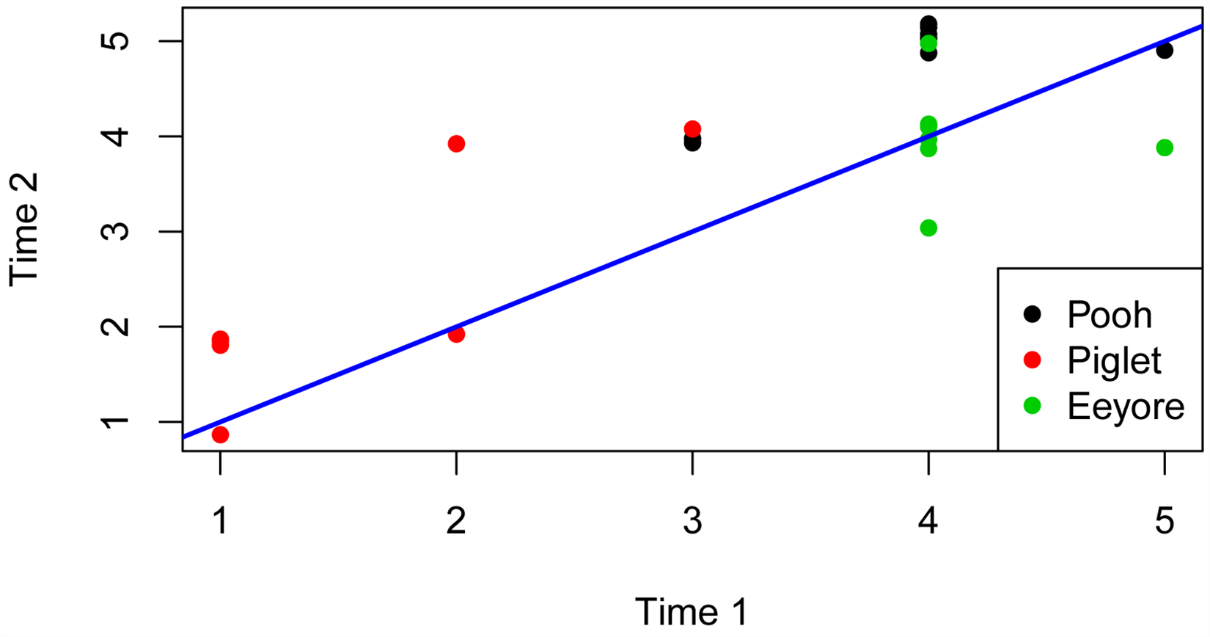#### Produce interaction plot with medians and confidence intervals

The following plot will show the interaction between Speaker and Time.  The median for each Speaker x Time combination is plotted, and the confidence intervals for each are shown with error bars.  In this case some of the medians and confidence limits are the same values, so some error bars are not visible.

The plot makes it easy to see the change in median score for each speaker from Time 1 to Time 2.

library(rcompanion)

Sum = groupwiseMedian(Likert ~ Speaker + Time,
data=Data,
conf       = 0.95,
R          = 5000,
percentile = TRUE,
bca        = FALSE,
digits     = 3)
Sum

library(ggplot2)

pd = position_dodge(.2)

ggplot(Sum, aes(x=Time,
y=Median,
color=Speaker)) +
geom_errorbar(aes(ymin=Percentile.lower,
ymax=Percentile.upper),
width=.2, size=0.7, position=pd) +
geom_point(shape=15, size=4, position=pd) +
theme_bw() +
theme(axis.title = element_text(face = "bold")) +

ylab("Median Likert score")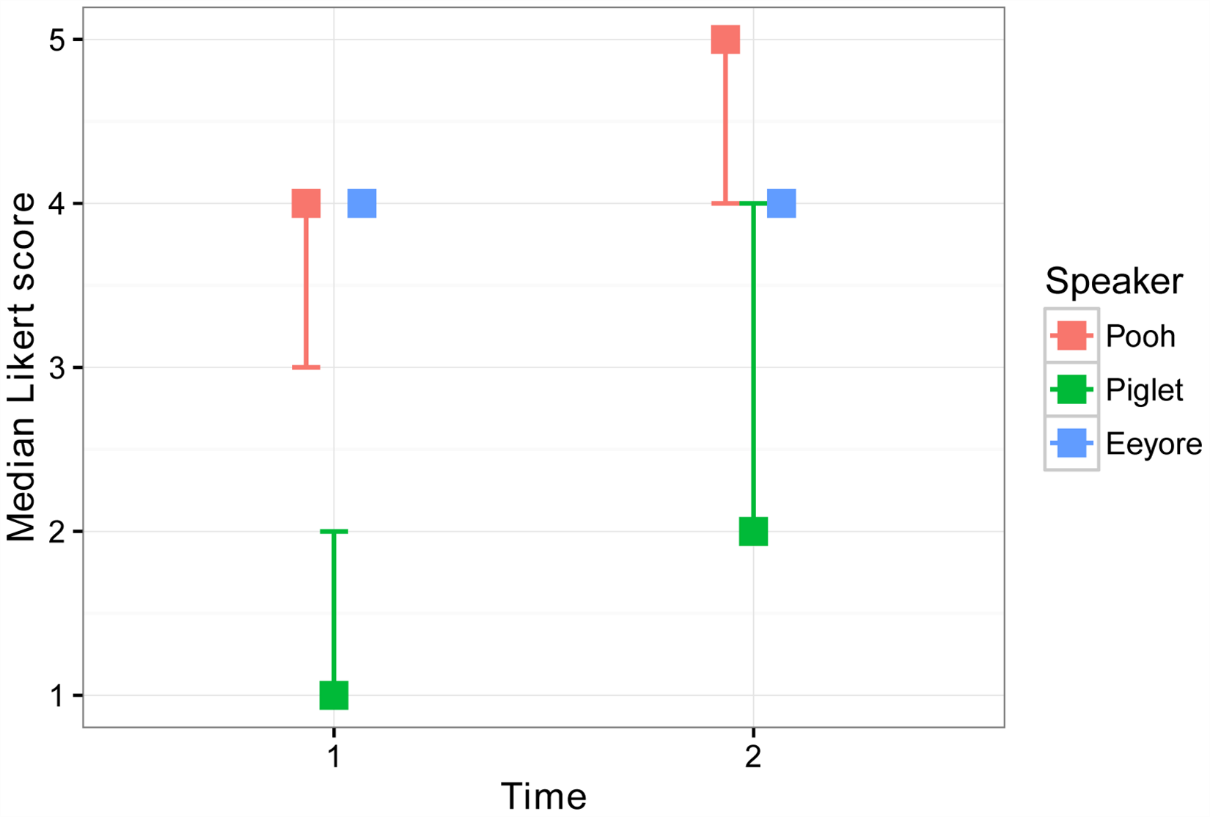#### Two-way repeated ordinal regression

In the model notation in the clmm function, here, Likert.f is the dependent variable and Speaker and Time are the independent variables.  The term Time:Spreaker adds the interaction effect of these two independent variables to the model.  Student is used as a blocking variable, and is entered as a random variable.  The data= option indicates the data frame that contains the variables.  For the meaning of other options, see ?clmm.

The p-values for the two main effects and the interaction effect is determined using the Anova function in the packages RVAideMemoire and car.

Here the threshold = "equidistant" option is used in order to avoid errors for this data set.  This option does not need to be used routinely.

##### Optional technical note

In the model below Student is treated as a simple random blocking variable, specified by (1|Student).  Note that in the data, students were lettered ax straight through the Speakers.  If the students had been lettered ah for each Speaker, but there were actually 24 students, we would need to tell the model that Student was nested within Speaker, by specifying that the random effect was (1|Speaker/Student), or that the random effect of interest was the interaction of Speaker and Student, by specifying (1|Speaker:Student).  This second specification is equivalent to that in the code below, with the original data, and will produce the same results.

I consider it a good practice to assign unique individuals with unique labels.  This practice is not always followed, but following the practice will avoid mis-specifying models.  In this case with 24 students, if they had been lettered ah for each Speaker, and the original model with the (1|Student) effect had been used, the results would be wrong because the model would treat Pooh’s student a as the same individual as Piglet’s student a.

##### Define model and conduct analysis of deviance

library(ordinal)

model = clmm(Likert.f ~ Time + Speaker + Time:Speaker + (1|Student),
data = Data,
threshold = "equidistant")

library(car)

library(RVAideMemoire)

Anova(model,
type = "II")

Analysis of Deviance Table (Type II tests)

LR Chisq Df Pr(>Chisq)
Time            9.774  1    0.00177 **
Speaker        34.485  2  3.249e-08 ***
Time:Speaker   28.202  2  7.517e-07 ***

##### p-value for model  and pseudo R-squared

In order to use the nagelkerke function for a clmm model object, a null model has to be specified explicitly.  (This is not the case with a clm model object).

We want the null model to have no fixed effects except for an intercept, indicated with a 1 on the right side of the ~.  But we also may want to include the random effects, in this case (1 | Student).

We can compare our full model against a model with an overall intercept and an intercept for each student.

Be sure that the threshold option in model.null is the same as in the original model.

model.null = clmm(Likert.f ~ 1 + (1 | Student),
data = Data
,
threshold = "equidistant")

library(rcompanion)

nagelkerke(fit  = model,
null = model.null
)

\$Pseudo.R.squared.for.model.vs.null
Pseudo.R.squared
Cox and Snell (ML)                   0.787504
Nagelkerke (Cragg and Uhler)         0.836055

\$Likelihood.ratio.test
Df.diff LogLik.diff  Chisq   p.value
-5     -37.172 74.344 1.275e-14

Another approach to determining the p-value and pseudo R-squared for a clmm model is comparing the model to a null model with only an intercept and neither the fixed nor the random effects.

model.null.2 = clm(Likert.f ~ 1,
data = Data
,
threshold = "equidistant")

library(rcompanion)

nagelkerke(fit  = model,
null = model.null.2
)

\$Pseudo.R.squared.for.model.vs.null
Pseudo.R.squared
Cox and Snell (ML)                   0.842667
Nagelkerke (Cragg and Uhler)         0.880526

\$Likelihood.ratio.test
Df.diff LogLik.diff  Chisq    p.value
-6     -44.385 88.771 5.4539e-17

##### Determine the effect of the random variable

The effect of the random variable (Student, in this case) can be determined by comparing the full model to a model with only the fixed effects (Speaker, Time, and Speaker:Time in this case).

First, model.fixed is defined, and then the two models are passed to the anova function.

Be sure that the threshold option in model.fixed is the same as in the original model.

model.fixed = clm(Likert.f ~ Time + Speaker + Time:Speaker,
data = Data,
threshold = "equidistant")

anova(model,
model.fixed)

Likelihood ratio tests of cumulative link models:

no.par     AIC  logLik LR.stat df Pr(>Chisq)
model.fixed      7 101.197 -43.598
model            8  78.268 -31.134  24.928  1   5.95e-07 ***

#### Post-hoc tests with lsmeans for multiple comparisons of groups

Be sure to read the Least Square Means for Multiple Comparisons chapter for correct interpretation of least square means.  For clmm model objects, the lsmean, SE, LCL, and UCL values should be ignored, unless specific options in lsmeans are selected.  See ?lsmeans::models for more information.

Because the interaction term in the model was significant, the group separations for the interaction is explored.

library(lsmeans)

marginal = lsmeans(model,
pairwise ~ Speaker + Time,
marginal

cld(marginal,
alpha   = 0.05,
Letters = letters,       ### Use lower-case letters for .group

Speaker Time    lsmean          SE df asymp.LCL asymp.UCL .group
Piglet  1    -36.08909 0.002735251 NA -36.09629 -36.08190  a
Piglet  2    -17.88982 0.003252687 NA -17.89838 -17.88127   b
Eeyore  2     17.46501 2.047095879 NA  12.07902  22.85100    c
Pooh    1     18.19627 0.002535027 NA  18.18960  18.20294    c
Eeyore  1     19.30751 2.030625019 NA  13.96486  24.65016    c
Pooh    2     36.72566 0.002910484 NA  36.71800  36.73332     d

Confidence level used: 0.95
Conf-level adjustment: sidak method for 6 estimates
P value adjustment: tukey method for comparing a family of 6 estimates
significance level used: alpha = 0.05

### Groups sharing a letter in .group are not significantly different

### Remember to ignore “lsmean”, “LCL”, “LCL”, and “UCL” with CLMM

##### Focusing on meaningful comparisons

Because we wanted to focus on the comparing each speaker at Time1 and Time 2, let’s rearrange the lsmeans cld table to focus on these comparisons.

With the results in this format, it is easy to see that the scores for both Pooh and Piglet improved from Time 1 to Time 2, but that the scores for poor Eeyore did not.

Speaker Time    lsmean          SE df  asymp.LCL asymp.UCL .group
Pooh    1     18.19627 0.002535027 NA  18.18960  18.20294    c
Pooh    2     36.72566 0.002910484 NA  36.71800  36.73332     d

Speaker Time    lsmean          SE df  asymp.LCL asymp.UCL .group
Piglet  1    -36.08909 0.002735251 NA -36.09629 -36.08190  a
Piglet  2    -17.88982 0.003252687 NA -17.89838 -17.88127   b

Speaker Time    lsmean          SE df  asymp.LCL asymp.UCL .group
Eeyore  1     19.30751 2.030625019 NA  13.96486  24.65016    c
Eeyore  2     17.46501 2.047095879 NA  12.07902  22.85100    c

#### Interaction plot with group separation letters

Group separation letters can be added manually to the plot of medians.##### Check model assumptions

At the time of writing, the nominal_test and scale_test functions don’t work with clmm model objects, so we will define a similar model with clm and no random effects, and test the proportional odds assumption on this model.

model.clm = clm(Likert.f ~ Time + Speaker + Time:Speaker + Student,
data = Data,
threshold = "equidistant")

Warning message:
(1) Hessian is numerically singular: parameters are not uniquely determined
In addition: Absolute convergence criterion was met, but relative criterion was not met.

###  The fitting of this model failed.  We’ll try a model without student.

model.clm = clm(Likert.f ~ Time + Speaker + Time:Speaker,
data = Data,
threshold = "equidistant")

nominal_test(model.clm)

Tests of nominal effects

formula: Likert.f ~ Time + Speaker + Time:Speaker
Df  logLik     AIC    LRT Pr(>Chi)
<none>          -43.598 101.197
Time          1 -43.149 102.298 0.8983  0.34325
Speaker       2 -40.053  98.106 7.0903  0.02886 *
Time:Speaker  5 -39.393 102.786 8.4110  0.13499

###  There is a violation of proportional odds assumption for this model.
###  Results from this model fitting may not be reliable.

scale_test(model.clm)

Warning messages:
1: (-1) Model failed to converge with max|grad| = 2.26258e-05 (tol = 1e-06)